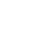# 单层钢结构厂房--檩条的设计

（一）隅撑的设计 隅撑按轴心受压构件设计。轴心力 N 按下式计算： 连接螺栓采用普通 C 级螺栓 M12 。 隅撑的计算长度取两端连接螺栓中心的距离： l 0 =633mm 。 选用 L50 × 4 ，截面特性： A=97.5px 2 ， I u =367.25px 4 ， W

##### （一）隅撑的设计A=97.5px 2 I u =367.25px 4 W u =104px 3 i u =48.5px i v =24.75px

λ u=l 0 / i u =633/19.4=32.6<[ λ ]=200

b 类截面，查表得ψ u =0.927

α y =0.6+0.0015 λ =0.696，满足要求。

### （二）檩条的设计

#### 1．  基本资料#### 2．  荷载及内力

P x =Psin α =0.153KN/m P y =Pcos α =1.528KN/m

M x =P y l 2 /8=1.528 × 6 2 /8=6.88KN · m

M y =P x l 2 /8=0.153 × 6 2 /32=0.17KN · m

#### 3．  截面选择及截面特性

(1)  选用 C180 × 70 × 20 × 2.2

I x =9372.5px 4 W x =1041.5px 3 i x =176.5px

I y =1224.25px 4 W ymax =579.75px 3 W ymin =250.5px 3 i y =63.74999999999999px ，χ 0 =52.75px(2) 受压板件的稳定系数

A ．腹板

k=7.8 6.29 ψ +9.78 ψ 2 =21.743

B ．上翼缘板

ψ = σ min / σ max =148.18/172.48=0.859> 1

k c =5.89 11.59 ψ +6.68 ψ 2 =0.863

(3) 受压板件的有效宽度

A ．腹板

k=21.743 k c =0.863 b=180mm c=70mm t=2.2mm ，σ 1 =172.48N/mm 2b c =b/(1 －ψ )=180/(1+0.915)=93.99mm

b/t=180/2.2=81.82

18 αρ =18 × 1.15 × 3.080=63.76 38 αρ =38 × 1.15 × 3.080=134.60b e1 =0.4b e =0.4 × 81.62=32.65mm b e2 =0.6b e =0.6 × 81.62=48.97mm

B ．上翼缘板

k=0.863 k c =21.743 b=70mm c=180mm ，σ 1 =172.48N/mm 2b e1 =0.4b e =0.4 × 57.05=22.82mm b e2 =0.6b e =0.6 × 57.05=34.23mm

C．下翼缘板(4) 有效净截面模量

93.99 81.62=12.37mm 同时在腹板的计算截面有一φ 13 拉条连接孔（距上翼缘板边缘 35mm ），孔位置与扣除面积位置基本相同。所以腹板的扣除面积按φ 13 计算，见图。有效净截面模量为：W enx /W x =0.915 W enymax /W ymax =0.973 W enymin /W ymin =0.972

#### 4 ．强度计算#### 5 ．挠度计算#### 6 ．构造要求

λ x =600/7.06=85.0<[ λ ]=200 ，满足要求

λ y =300/2.55=117.6<[ λ ]=200 ，满足要求

### （三）墙梁设计

#### 2 ．荷载计算

(1)  墙梁采用冷弯薄壁卷边 C 型钢 160 × 60 × 20 × 2.5 ，自重 g=7kg/m

(2)  墙重 0.22KN/m 2

(3)  风荷载

q x =1.2 × 0.07=0.084KN/m q y = 1.1 × 0.473 × 1.5 × 1.4= 1.093KN/m

#### 3 ．内力计算

M x =0.084 × 6 2 /8=0.378KN · m M y =1.093 × 6 2 /8=4.919KN · m

#### 4 ．强度计算

W xmax =486.75px 3 W min =216.5px 3 W y =900.5000000000001px 3 Iy =7203.25px 4

W enx =0.9 W x W eny =0.9 W y#### 5 ．挠度计算### （四）山墙抗风柱设计

#### 2．  荷载计算

(1)  抗风柱选用焊接工字钢 300 × 200 × 6 × 10 ，自重 g 1 =44.6kg/m

(2)  墙梁及其支撑构件重量取 g 2 =7kg/m

(3)  风荷载：按 CECS102:2002 中的围护结构计算。

ω k = μ s μ z ω 0 ，μ s = 1.0(+1.0) ，ω 0 =1.05 × 0.45=0.473KN/m 2

q z =1.2 × (0.07 × 6 × 3+44.6 × 6.274 × 10 -2 )=4.87KN

q y =1.4 × 1.0 × 1.0 × 0.473 × 6=3.97KN/m

#### 3．  内力计算

N=4.87KN M=1/8 × 3.97 × 6.274 2 +0.35=19.88KN · m

#### 4．  验算构件的局部稳定性#### 5．  强度验算

I y =33350px 4 W y =3335px 3 i y =121.24999999999999px#### 6．  验算弯矩作用平面内的稳定性

λ =48.5 b 类截面，查表得ψ x =0.863=30.85N/mm 2 2 ，满足要求。

#### 7．  验算弯矩作用平面外的稳定性

l 0y =3000mm ，λ y = l 0y / i y =3000/48.5=61.9<[ λ ]=150 b 类截面，查表得ψ y =0.797，η =1.0 ，β tx =1.0

=32.97N/mm 2 2 ，满足要求。

#### 8．  挠度验算#### 9．  柱脚设计

400 × 300 × 20 ；锚栓采用 2M20 ，平面布置如图。

### （五）柱间支撑的设计

1．柱间支撑的布置如图

2．  柱间支撑为斜杆，采用带张紧装置的十字交叉圆钢支撑。直杆用檩条兼用，因檩条留有一定的应力裕量，根据经验及类似工程，不再作压弯杆件的刚度及承载力验算。

3．  柱间支撑荷载及内力按一半山墙面作用风载的 1/3 考虑节点荷载标准值为：

F wk =1/3 × 1/2 × 38.70=6.45KN

4．  斜杆截面设计及强度验算

2

### （六）屋面支撑设计

1．  屋面支撑布置2．  屋面支撑荷载及内力

3 ．斜杆截面设计及强度验算

• 收藏0

• 打赏0

#### 钢结构##### 相关标签